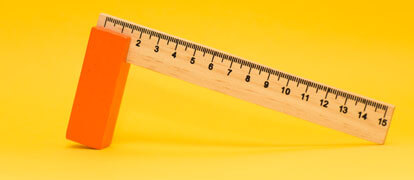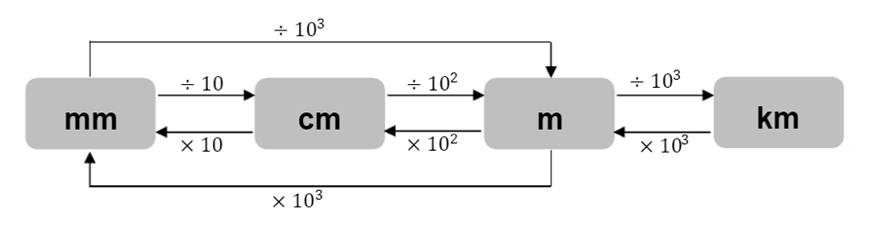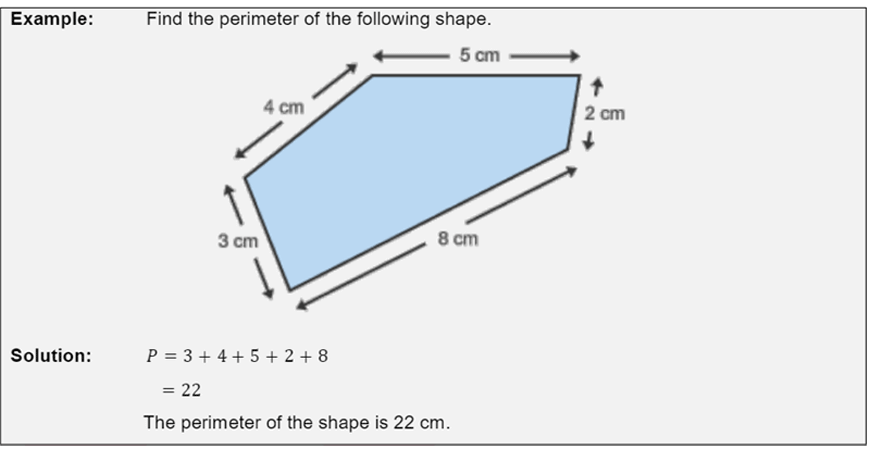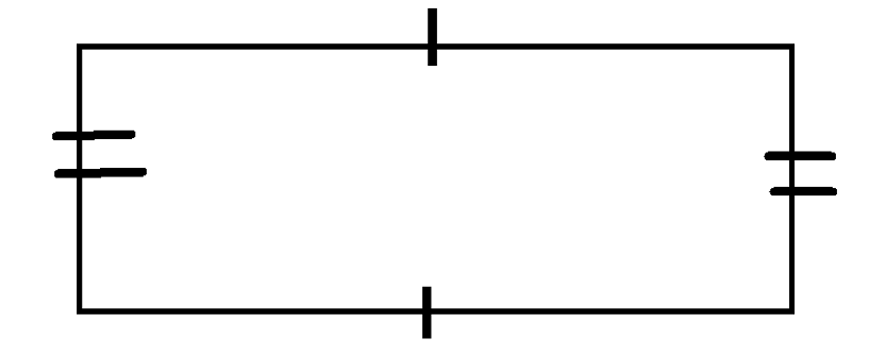# Part 5: Length

In this article, we will go through converting units over the same system and how to calculate perimeters of shapes.Length is an essential foundation that is need in other numerous Maths topics, like area. So, it is crucial that you know and understand it. Read on to find out more about Year 7 length.

## Syllabus

 Stage 4 NESA Syllabus Syllabus Explanation Find perimeters of parallelograms, trapeziums, rhombuses and kites (ACMMG196) You are able to calculate the total length of a shape, given any side. You are also able to convert units to figure out the perimeter.

## Outline

In this section, students are exposed to questions involving the usage of length.

In this guide, we will be converting different length measurements across the metric system.

E.g. metres to kilometres, calculating the perimeter of 2-dimensional shapes and also examples of some real-life application and worded questions involving length.

Length is the measurement or extent of something from end to end.

This is quite a simple concept. So, you would have already experienced basic length equations already in general conversation.

For example, these all require knowledge of length:

• Parents talking about the mileage on their car
• Height of your growth over the past year
• Determining the distance from home to school all require knowledge of length

## Assumed knowledge

However, length may sometimes be paired with other mathematical concepts to make a question harder. In these cases, knowledge of the other concepts are also required to answer the question.

For example, if you had to calculate the perimeter of a square, and you were only given the length of one side… you need to know that all sides of a square are equal.

## Converting units over the same system

### Quick indices recap:

Note that prefixes may apply to a variety of mathematical concepts, not just length.

Eg. A millilitre is one thousandth of a litre, just as a millimetre is a thousandth of a metre.

This standardised form makes the metric system arguably superior to the older imperial system used in the US.

For example:

\begin{align*}
10^{3} &= 10 \times 10 \times 10 = 1000 \\
10^{-2} &= 10 = \frac{1}{10^{2}} = \frac{1}{10 \times 10} = \frac{1}{100}
\end{align*}

### Converting units

There are many different units for measuring length. Centimetre, millimetre and kilometre are just some of them.

In general use, depending on the circumstance, one particular unit may be favoured over another.

Eg. Longer distances such as the distance from Sydney to Melbourne, would be calculated in kilometres, while a small distance like the length of a caterpillar may well be calculated in millimetres.

For a measurement like height, a mixture of both metres and centimetres may be used.

Eg. You might say “Sean is 1 metre 54″. This implies that his height is 1 metre and 54 centimetres.

Thus, it is important to know the conversions of different units over the metric system.

Lucky for us, the system for length which Australia uses is the metric system.

It is easily convertible between units as it is all in powers of 10.

Therefore, converting only requires a knowledge of some mathematical prefixes.

Some common prefixes include:

• Milli – one thousandth or $$\frac{1}{1000} = 10^{-3}$$
• Centi – one hundredth or $$\frac{1}{100} = 10^{-2}$$
• Kilo – one thousand or $$1000 = 10^{3}$$
• Mega – one million or $$1 000 000 = 10^{6}$$

A conversion flowchart is a helpful way to see the relationship between units of different prefixes.To convert from mm to cm you would follow the arrow from mm to cm and see that all you have to do is divide by $$10$$.

Note: To do a conversion in the opposite direction, all you have to do is use the inverse operation. That is, changing multiplication to division, or division to multiplication.

For example:

To convert metres to kilometres, we follow the arrow and divide by $$10^{3}$$.

Conversely to change from kilometres to metres, we multiply by $$10^{3}$$.

To go from metres to kilometres to metres, is just metres to metres… which obviously is nothing.

So, we’d expect to cancel out whatever operations we perform.

Eg. $$\times 10^{3} \div 10^{3} = \times \frac{10^{3}}{10^{3}} = \times 1$$

Multiplying anything by $$1$$ does nothing to the value.

Example

1. Arrange the following lengths in ascending order:

\begin{align*}
\color{blue}{320 000mm, 3km, 3289cm, 396.8m}
\end{align*}

Solution:

To start, we convert everything into the same unit.

For this particular example, we will be picking the metre as our choice.

This gives:

\begin{align*}
\color{blue}{320 000 \div 10^{3}, 3 \times 10^{3}, 3289 \div 10^{2}, 396.8} \\
\color{blue}{320m, 3000m, 32.89m, 396.8m}
\end{align*}

Now we rearrange to ascending order:

\begin{align*}
\color{blue}{32.89m, 320m, 396.8m, 3000m}
\end{align*}

To finish off, we must convert the units back to their original form, but keep desired ordering

\begin{align*}
\color{blue}{3289cm, 320 000mm, 396.8m, 3km}
\end{align*}

These lengths are now arranged in ascending order as requested

## Perimeter

The perimeter is the added length of a continuous line forming the boundary of a closed geometrical figure.

What this means simply, is that perimeter is the summed length of all the sides of a particular shape.

Let’s look at a simple example,Note: Some harder questions may rely on your knowledge of geometrical figures.

For example, a square and rhombus, both consist of 4 equal sides. For triangles, equilateral triangles have 3 equal sides, isosceles have 2, while scalene have none.

A dash or double dash on a geometrical diagram indicates that a length is equal to another.The single dash indicates that the top and bottom side are equal.

The double dashes say that the left and right sides are equal.

If we are given that the corner angles are all right angles, we can also positively confirm that this shape is a rectangle.

Rectangles have equal opposite sides and all their interior angles are right angles.

Other questions may require use of basic algebra.

In these cases, always create a pronumeral and see what you can solve from there.

Examples

1. The side lengths of an equilateral triangle are $$25cm$$. Find its perimeter.

Solution:

Equilateral means all three sides are equal.

\begin{align*}
P = 25 \times 3 \\
P = 75cm
\end{align*}

2. One side of a rectangle is $$14cm$$ longer than the other side. If the perimeter of said rectangle is $$56cm$$, what are the lengths of the sides?

Solution:

Let us first set the shorter side to pronumeral $$x$$, the longer side is therefore, $$14 + x$$

\begin{align*}
56 &= x + x + (14+x) + (14+x) \\
56 &= 2x + 28 + 2x \\
56 &= 4x + 28 \\
28 &= 4x \\
x &= 7
\end{align*}

Thus, we can see that the shorter side is $$7$$, and the longer side being $$14 + 7$$ must now be $$21$$. Therefore, the lengths of the sides are $$7cm$$ and $$21cm$$.

3. A square paddock has a side length of $$135m$$. If fencing costs $$1250$$ per kilometre, what is the cost of fencing the paddock.

Solution:

What we do first, is put everything in the same units.

That is, we will change 1250 per kilometre, into dollars per metre. From here, we will then calculate the total perimeter of the paddock. Then simply multiply to the cost per metre with the required metres we need to fence. \begin{align*}1250/kilometre \ & = 1250/1000metres \\
&= \$1.25 / metre \\
P &= 4 \times 135 \\
P &= 540m
\end{align*}

Cost will be $$540 \times 1.25 = 675$$

Therefore, the cost of fencing the paddock is $$675$$, quite cheap considering its size!

## Summary

To succeed with length-based questions, you should practice:

• Converting across the metric system
• Various methods for calculating the perimeter of geometrical shapes.

Harder questions will require adequate knowledge of:

• Other mathematical concepts
• Knowing length

This will form a strong base for the further high school mathematics you will encounter within the coming years.

Understanding length is also essential before attempting to learn area, another core topic of Year 7 Mathematics.

## Master the basics to excel in Maths!

Improve your problem solving skills and gain a thorough understanding of basic Maths principles with Matrix. Learn more about our Year 7 Maths course now!

© Matrix Education and www.matrix.edu.au, 2023. Unauthorised use and/or duplication of this material without express and written permission from this site’s author and/or owner is strictly prohibited. Excerpts and links may be used, provided that full and clear credit is given to Matrix Education and www.matrix.edu.au with appropriate and specific direction to the original content.